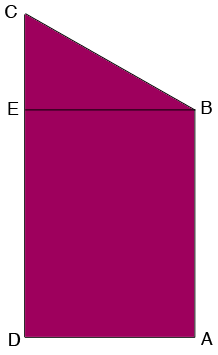SEARCH HOMEMath Central Quandaries & QueriesQuestion from joanna: left vertical measurement 2560mm right vertical measurement 1850mm base horizontal measurement 1750mm question - what will the 4th measurement be please. using a scale drawing I make it approx 1900mm but require an accurate measurement regards JoannaHi Joanna,

You use the terms horizontal and vertical so I am assuming the the angles $CDA$ and $DAB$ in my rough sketch are right angles.I drew the line $BE$ parallel to $DA$ and hence angle $CEB$ is a right angle and hence we can use Pythagoras Theorem on the triangle $CEB.$ thus

$|BC|^2 = |CE|^2 + |EB|^2 = (2560 - 1850)^2 + 1750^2 \;mm^2$

and hence

$|BC| = \sqrt{3566600} = 1888.54 \;\; mm$

PennyMath Central is supported by the University of Regina and The Pacific Institute for the Mathematical Sciences.